By going through these CBSE Class 12 Maths Notes Chapter 11 Three Dimensional Geometry, students can recall all the concepts quickly.

## Three Dimensional Geometry Notes Class 12 Maths Chapter 11

1. Direction cosines of a line:
Let AB be a line in space.
Through O, draw a line OP parallel to AB. Let OP makes angles α, β, and γ with OX, OY, and OZ respectively.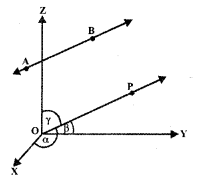Cosines of the angles α, β, and γ,
i.e., cos α, cos β, and cos γ are known as the direction cosines of line AB.
Let l = cos α, m = cos β, n = cos γ
⇒ l, m, n are the direction cosines of the line AB Let us consider the ray BA. OQ is drawn parallel to BA. Now, OQ makes angles n – α, n – β, and n – γ with coordinates axes OX, OY, and OZ respectively.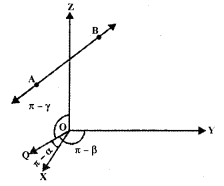∴ Direction cosines of BA are cos(π – α), cos (π – β) and cos (π – γ),
i.e., – cos α, – cos β, – cos γ or -l, -m, -n.

→ Relation between l, m, and n
AB is any ray having direction cosines l, m, n. Now OP is drawn parallel to AB, where P is the point (x, y, z). Let PM be drawn perpendicular to OY.In ΔOPM OP = r (say). PM⊥OY.
∴ ∠PMO = 90°
Also, ∠POM = β.
∴ $$\frac{\mathrm{OM}}{\mathrm{OP}}$$ = β
∴ $$\frac{y}{r}$$ = m,
∴ y = rm

Similarly, x = rl and z = rn.
Now, OP2 = x2 + y2 + z2
r2 = (rl)2 + (rm)2 + (rn)2
or
r2 = r2(l2 + m2 + n2)
⇒ l2 + m2 + n2 = 1.

→ Direction Ratios of a line
Definition: The numbers which are proportional to the direction cosines of a line are known as direction ratios of the line.

Let Z, m, n be the direction cosines of a line.
Multiplying each by r, we ger rl, rm, rn the direction ratios.
Let rl = a,rm = b and rn – c.
r2l2 + r2m2 + r2n2 = a2 + b2 + c2
∴ r2(l2 + m2 + n2) = a2 + b2 + c2
∵ l2 + m2 + n2 = 1
∴ l = $$\frac{a}{r}=\frac{a}{\sqrt{a^{2}+b^{2}+c^{2}}}$$

Similarly, m = $$\frac{b}{\sqrt{a^{2}+b^{2}+c^{2}}}$$ and n = $$\frac{c}{\sqrt{a^{2}+b^{2}+c^{2}}}$$

Thus, if a, b and c are the direction ratios of a line, the direction cosines are $$\frac{a}{\sqrt{a^{2}+b^{2}+c^{2}}}$$, $$\frac{b}{\sqrt{a^{2}+b^{2}+c^{2}}}$$ and $$\frac{c}{\sqrt{a^{2}+b^{2}+c^{2}}}$$.

→ Direction cosines of the line passing through the points P(x1, y1, z1) and Q(x2, y2, z2)
Direction ratios of the line PQ are x2 – x1, y2 – y1, z2 – z1
∴ PQ = $$\sqrt{\left(x_{2}-x_{1}\right)^{2}+\left(y_{2}-y_{1}\right)^{2}+\left(z_{2}-z_{1}\right)^{2}}$$
∴Direction cosines of PQ are $$\frac{x_{2}-x_{1}}{\mathrm{PQ}}$$, $$\frac{y_{2}-y_{1}}{\mathrm{PQ}}$$, $$\frac{z_{2}-z_{1}}{P Q}$$

2. Angle between the two lines
1. Let l1, m1, n1, and l2, m2, n2 be the direction cosines of the lines OP and OQ.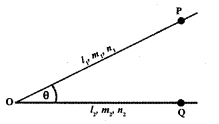The angle θ between these lines is given by
cos θ = l1l2 + m1m2 + n1n2
and sin θ = $$\sqrt{\left(m_{1} n_{2}-m_{2} n_{1}\right)^{2}+\left(n_{1} l_{2}-n_{2} l_{1}\right)^{2}+\left(l_{1} m_{2}-l_{2} m_{1}\right)^{2}}$$

2. If a1, b1, c1 and a2, b2, c2 are the direction ratios of two lines, then3. Two lines are perpendicular to each other,
if θ = $$\frac{π}{2}$$ ⇒ cos θ = cos $$\frac{π}{2}$$ = 0
⇒ l1l2 + m1m2 + n1n2 = 0
or
a1a2 + b1b2 + c1c2 = 0

4. When the lines are parallel
θ = 0 ⇒ sin θ = sin 0 = 0.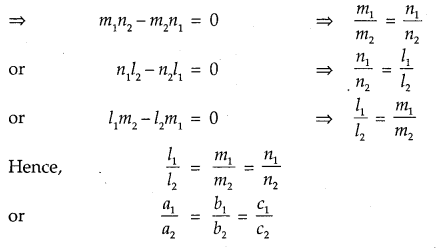STRAIGHT LINE
3. Equation of a line through a given point:
(a) Let the line passes through $$\vec{a}$$ and is parallel to vector $$\vec{b}$$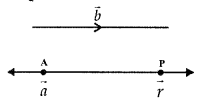Then, the equation of the line is
$$\vec{r}$$ – $$\vec{a}$$ = λ$$\vec{b}$$
or
$$\vec{r}$$ = $$\vec{a}$$ + λ$$\vec{b}$$

(b) Let the point A be (x1, y1, z1) and a, b, c are the direction ratios of the line. The equation of the line is
$$\frac{x-x_{1}}{a}=\frac{y-y_{1}}{b}=\frac{z-z_{1}}{c}$$
If l, m, n are the direction cosines, then equation of the line is
$$\frac{x-x_{1}}{l}=\frac{y-y_{1}}{m}=\frac{z-z_{1}}{n}$$

4. Equation of the line passing through two points:
(a) Let $$\vec{a}_{1}$$, $$\vec{a}_{2}$$ be the position vectors of two points P and Q respectively.
⇒ $$\vec{b}$$ = $$\vec{a}_{1}$$ – $$\vec{a}_{2}$$∴ Equation of PQ is
$$\vec{r}$$ = $$\vec{a}$$ + λ($$\vec{a}_{2}$$ – $$\vec{a}_{1}$$)

(b) Direction ratios of the line passing through P(x1, y1, z1) and Q(x2, y2, z2) are x2 – x1, y2 – y1 and z2 – z1
∴ Equation of the line PQ is
$$\frac{x-x_{1}}{x_{2}-x_{1}}=\frac{y-y_{1}}{y_{2}-y_{1}}=\frac{z-z_{1}}{z_{2}-z_{1}}$$

5. Angle between two straight lines: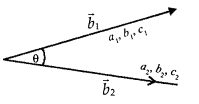(a) Let the two lines be
$$\vec{r}$$ = $$\vec{a}_{1}$$ + λ$$\vec{b}_{1}$$
$$\vec{r}$$ = $$\vec{a}_{2}$$ + λ$$\vec{b}_{2}$$
If θ be the angle between them, then
cos θ = $$\frac{\vec{b}_{1} \vec{b}_{2}}{\left|\vec{b}_{1}\right|\left|\vec{b}_{2}\right|}$$

(b) Let the lines be
$$\frac{x-x_{1}}{a_{1}}=\frac{y-y_{1}}{b_{1}}=\frac{z-z_{1}}{c_{1}}$$
and $$\frac{x-x_{2}}{a_{2}}=\frac{y-y_{2}}{b_{2}}=\frac{z-z_{2}}{c_{2}}$$
i.e., a1, b1, c1 and a2, b2, c2 are the direction ratios of the lines.
∴ cos θ = $$\frac{a_{1} a_{2}+b_{1} b_{2}+c_{1} c_{2}}{\sqrt{a_{1}^{2}+b_{1}^{2}+c_{1}^{2}} \sqrt{a_{2}^{2}+b_{2}^{2}+c_{2}^{2}}}$$
If l1, m1, n1 and l2, m2, n2 are the direction cosines, then
cos θ = l1l2 + m1m2 +n1n2

6. Shortest Distance:
(a)Let $$\vec{r}$$ = $$\vec{a}_{1}$$ + λ$$\vec{b}_{1}$$ and
$$\vec{r}$$ = $$\vec{a}_{2}$$ + λ$$\vec{b}_{2}$$ be the two non-intersecting lines.
The shortest distance between the given lines = $$\left|\frac{\left(\vec{b}_{1} \times \vec{b}_{2}\right) \cdot\left(\vec{a}_{2}-\vec{a}_{1}\right)}{\left|\vec{b}_{1} \times \vec{b}_{2}\right|}\right|$$

(b) Let the lines be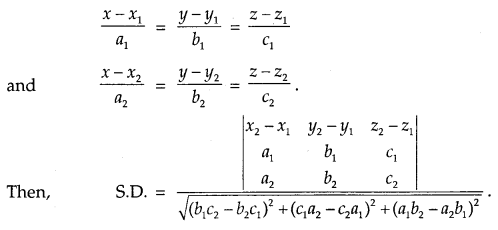If the lines are intersecting, then lines are coplanar.
⇒ S.D. = 0
⇒ ($$\vec{b}_{1} \times \vec{b}_{2}$$) – ($$\vec{a}_{1} \times \vec{a}_{2}$$) = 0
or
$$\left|\begin{array}{ccc} x_{2}-x_{1} & y_{2}-y_{1} & z_{2}-z_{1} \\ a_{1} & b_{1} & c_{1} \\ a_{2} & b_{2} & c_{2} \end{array}\right|$$ = 0

7. Distance between parallel lines:
Let the parallel lines be
$$\vec{r}$$ = $$\vec{a}_{1}$$ + λ$$\vec{b}_{1}$$ and
$$\vec{r}$$ = $$\vec{a}_{2}$$ + μ$$\vec{b}_{2}$$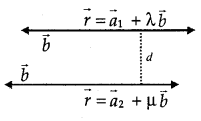Shortest distance d between these lines is given by,
d = $$\left|\frac{\vec{b} \times\left(\vec{a}_{1}-\vec{a}_{2}\right)}{|\vec{b}|}\right|$$

PLANES
8. Different forms of equations of a plane:
1. Normal Form.
(a) Let the plane ABC be at a distance d from the origin. ON is the normal to the plane in the directon n̂. Equation of the plane is $$\vec{r}$$. n̂ = d.(b) If l, m, n are the direction cosines of the normal to the plane which is at distance d from the origin. The equation of the plane is lx + my + nz = d.
However, general form of the equation of a plane are $$\vec{r}$$ .$$\overrightarrow{\mathrm{N}}$$ = D and Ax + By + Cz + D = 0.

2. (a) Let the plane passes through a point A and let it perpendicular to the vector $$\overrightarrow{\mathrm{N}}$$
∴ Equation of the plane
($$\vec{r}$$ – $$\vec{a}$$).$$\overrightarrow{\mathrm{N}}$$ = 0.(b) If a plane passes through (x1, y1, z1) and perpendicular to the line with direction ratios a, b, c the equation of the plane is
a(x – x1) + b(y – y1)+c(z – z1) =0

3. Equation of the plane passing through three points.
(a) Let the three points be A, B and C whose position vectors be, $$\vec{a}$$, $$\vec{b}$$ and $$\vec{c}$$
The equation of the plane is
$$(\vec{r}-\vec{a}) \cdot[(\vec{b}-\vec{a}) \times(\vec{c}-\vec{a})]$$ = 0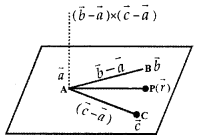(b) Let the three points through which the plane is passing be A(x1, y1, z1), B(x2, y2, z2), and C(x3, y3, z3).
Then, equation of the plane is
$$\left|\begin{array}{llll} x & y & z & 1 \\ x_{1} & y_{1} & z_{1} & 1 \\ x_{2} & y_{2} & z_{2} & 1 \\ x_{3} & y_{3} & z_{3} & 1 \end{array}\right|$$ = 0
or
$$\left|\begin{array}{ccc} x-x_{1} & y-y_{1} & z-z_{1} \\ x_{2}-x_{1} & y_{2}-y_{1} & z_{2}-z_{1} \\ x_{3}-x_{1} & y_{3}-y_{1} & z_{3}-z_{1} \end{array}\right|$$ = 0

4. Intercepts form of the equation of the plane
Let the plane make the intercepts a, b, and c on coordinate axes OX, OY, and OZ respectively.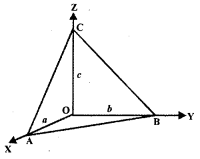Then, the equation of the plane is
$$\frac{x}{a}+\frac{y}{b}+\frac{z}{c}$$ = 1

9. Plane passing through the intersection of the two planes:
Let the equation of two planes be
$$\vec{r}$$. $$\vec{n}_{1}$$ = d1 and
$$\vec{r}$$ .$$\vec{n}_{2}$$ = d2

The equation of the plane passing through the line of intersection of the given planes is
($$\vec{r}$$. $$\vec{n}_{1}$$ – d1) + λ($$\vec{r}$$ .$$\vec{n}_{2}$$ – d2) = 0
or
$$\vec{r}$$ .($$\vec{n}_{1}$$ + λ$$\vec{n}_{2}$$) = (d1 + λd2)

(b) Equation of the plane passing through the line of intersection of the planes
a1x + b1y + c1z + d1 = 0 and a2x + b2y + c2z + d2 = 0 is (a1x + b1 y + c1z + d1 + λ(a2x + b2y + c2z + d2) = 0
or
(a1 + λa2)x + (b1 + λb2)y + (c1 + λc2)z + d1 + λd2 = 0,
where λ is determined according to the given condition.

10. Coplanarity of two lines: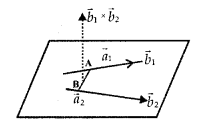(a) The two lines
$$\vec{r}$$ = $$\vec{a}_{1}$$ + λ$$\vec{b}_{1}$$ and
$$\vec{r}$$ = $$\vec{a}_{2}$$ + λ$$\vec{b}_{2}$$ intersect each other, if $$\left(\vec{a}_{2}-\vec{a}_{1}\right) \cdot\left(\vec{b}_{2} \times \vec{b}_{1}\right)$$ = 0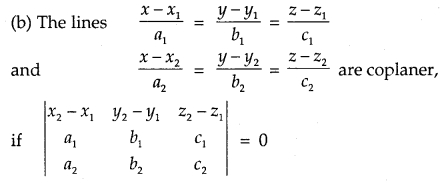11. The angle between the planes:
Definition : The angle between the two planes is the angle between their normals. The angle 0 between the planes
$$\vec{r}$$ = $$\vec{n}_{1}$$ = d1 and $$\vec{r}$$ = $$\vec{n}_{1}$$ = d2 is given by cos θ = $$\frac{\left|\vec{n}_{1} \cdot \vec{n}_{2}\right|}{\left|\vec{n}_{1}\right|\left|\vec{n}_{2}\right|}$$

(b) If the planes are
a1x + b1y + C1z + d1 = 0
a2x + b2y + c2z + d2 = 0, then the angle θ between these planes is given by
cos θ = $$\frac{a_{1} a_{2}+b_{1} b_{2}+c_{1} c_{2}}{\sqrt{a_{1}^{2}+b_{1}^{2}+c_{1}^{2}} \sqrt{a_{2}^{2}+b_{2}^{2}+c_{2}^{2}}}$$

(c) The planes are perpendicular to each other, if θ = $$\frac{π}{2}$$
∴ cos $$\frac{π}{2}$$ = 0 ⇒ $$\vec{n}_{1}$$.$$\vec{n}_{2}$$ = 0
or
a1a2 + b1b2 + c1c2 = 0

(d) The planes a1x + b1y +c1z + d1 = 0
and a2x + b2y + c2z + d2 = O
are parallel, if $$\frac{a_{1}}{a_{2}}=\frac{b_{1}}{b_{2}}=\frac{c_{1}}{c_{2}}$$.

(e) Equation of the plane parallel to
$$\vec{r} \cdot \vec{n}$$ = d is $$\vec{r} \cdot \vec{n}$$ = λ
Plane parallel to
ax + by + cz + d = 0 is ax + by + cz + λ = 0.

12. Distance of a point from th plane:
(a) Let the plane be $$\vec{r} \cdot \vec{n}$$ = d.
∴ Perpendicular distance of the point $$\vec{a}$$ from the plane
=|d – $$\vec{a} \cdot \hat{n}$$|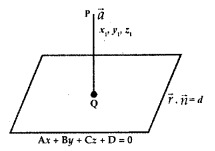(b) Let the equation of the plane
be Ax + By + Cz + D= O.
The distance of the point (x1, y1, z1) from this plane
= $$\left|\frac{A x_{1}+B y_{1}+C z_{1}+D}{\sqrt{A^{2}+B^{2}+C^{2}}}\right|$$

13. Angle between a line and a plane:
Definition: The angle between a line and plane is said to be the complement of the angle between the line and the normal to the plane.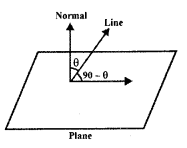(a) Let the line and plane be
$$\vec{r}$$ = $$\vec{a}$$ + λ$$\vec{b}$$ and $$\vec{r} \cdot \vec{n}$$ = d.

If θ be the angle between the plane and the line, then
sin θ = $$\frac{\vec{b} \cdot \vec{n}}{|\vec{b}||\vec{n}|}$$

(b) ¡et the line and the plane be $$\frac{x-x_{1}}{a}=\frac{y-y_{1}}{b}=\frac{z-z_{1}}{c}$$ and Ax + By + Cz + D = O.
The angle Φ between them is given by
sin Φ = $$\frac{a \cdot \mathrm{A}+b \cdot \mathrm{B}+c \cdot \mathrm{C}}{\sqrt{a^{2}+b^{2}+c^{2}} \sqrt{\mathrm{A}^{2}+\mathrm{B}^{2}+\mathrm{C}^{2}}}$$.

1. IMPORTANT RESULT

l2 + m2 + n2 = 1, where < l, m, n > are direction-cosines of a st. line.

2. DIRECTION-RATIOS

The direction-ratios of the line joining of the points (x1, y1, z1) and (x2, y2, z2) are :
<x2-x1, y2-y1 ,z2-z1 >.

3. (i) Angle between two lines. The angle between two lines having direction-cosines
< l1 m1 n1 > and < l2, m2, n2 > is given by :
cos θ = |l1l2+ m1m2 + n1n2|.

(ii) The lines are:
(a) perpendicular l1l2+ m1m2 + n1n2 = 0.
(b) parallel iff l1 = l2, m1 = m2, + n1 = n

4. SHORTEST DISTANCE

The shortest distance between two lines :
$$\vec{r}=\overrightarrow{a_{1}}+\lambda \overrightarrow{b_{1}} \text { and } \vec{r}=\overrightarrow{a_{2}}+\mu \overrightarrow{b_{2}} \text { is }\left|\frac{\left(\overrightarrow{b_{1}} \times \overrightarrow{b_{2}}\right) \cdot\left(\overrightarrow{a_{2}}-\overrightarrow{a_{1}}\right)}{\left|\overrightarrow{b_{1}} \times \overrightarrow{b_{2}}\right|}\right|$$

5. EQUATIONS OF PLANES

(i) Equation of a plane, which is at a distance ‘p’ from the origin and perpendicular to the unit
vector $$\hat{n}$$ is $$\vec{r} \cdot \hat{n}=p$$

(ii) General Form. The general equation of first degree i.e.ax + by + cz + d- 0 represents a plane,

(iii) One-point Form. The equation of a plane through (*,, y,, z,) and having <a,b,c> as direction-
ratios of the normal is a (x – x1) + b (y – y1) + c (z – z1) = 0.

(iv) Three-point Form. The equation of the plane through (x1, y1, z1), (x2, y2, z2) and (x3, y3, z3) is:
$$\left|\begin{array}{ccc} x-x_{1} & y-y_{1} & z-z_{1} \\ x-x_{2} & y-y_{2} & z-z_{2} \\ x-x_{3} & y-y_{3} & z-z_{3} \end{array}\right|=0$$

(v) Intercept Form. Equation of the plane, which cuts off intercepts a, b, c on the axes, is :
$$\frac{x}{a}+\frac{y}{b}+\frac{z}{c}$$ = 1

6. ANGLE BETWEEN TW O PLANES
The angle between the planes :
a1x + b1y + c1z + d1 = 0 and a2x + b2y + c2z + d2 = 0 is given by :
cos θ = $$\frac{\left|a_{1} a_{2}+b_{1} b_{2}+c_{1} c_{2}\right|}{\sqrt{a_{1}^{2}+b_{1}^{2}+c_{1}^{2}} \sqrt{a_{2}^{2}+b_{2}^{2}+c_{2}^{2}}}$$

7. BISECTING PLANES
The equations of the planes bisecting the planes :
a1x + b1y + c1z + d1 = 0 and a2x + b2y + c2z + d2 = 0 are:
$$\frac{a_{1} x+b_{1} y+c_{1} z+d_{1}}{\sqrt{a_{1}^{2}+b_{1}^{2}+c_{1}^{2}}}=\pm \frac{a_{2} x+b_{2} y+c_{2} z+d_{2}}{\sqrt{a_{2}^{2}+b_{2}^{2}+c_{2}^{2}}}$$